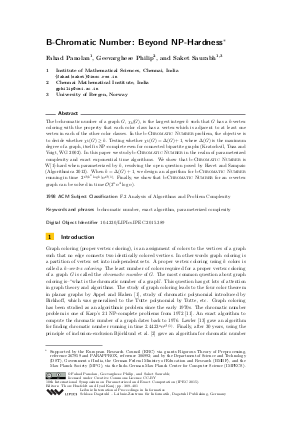Document# B-Chromatic Number: Beyond NP-Hardness

### Authors Fahad Panolan, Geevarghese Philip, Saket Saurabh## File

LIPIcs.IPEC.2015.389.pdf
• Filesize: 0.49 MB
• 13 pages

## Cite As

Fahad Panolan, Geevarghese Philip, and Saket Saurabh. B-Chromatic Number: Beyond NP-Hardness. In 10th International Symposium on Parameterized and Exact Computation (IPEC 2015). Leibniz International Proceedings in Informatics (LIPIcs), Volume 43, pp. 389-401, Schloss Dagstuhl - Leibniz-Zentrum für Informatik (2015)
https://doi.org/10.4230/LIPIcs.IPEC.2015.389

## Abstract

The b-chromatic number of a graph G, chi_b(G), is the largest integer k such that G has a k-vertex coloring with the property that each color class has a vertex which is adjacent to at least one vertex in each of the other color classes. In the B-Chromatic Number problem, the objective is to decide whether chi_b(G) >= k. Testing whether chi_b(G)=Delta(G)+1, where Delta(G) is the maximum degree of a graph, itself is NP-complete even for connected bipartite graphs (Kratochvil, Tuza and Voigt, WG 2002). In this paper we study B-Chromatic Number in the realm of parameterized complexity and exact exponential time algorithms. We show that B-Chromatic Number is W-hard when parameterized by k, resolving the open question posed by Havet and Sampaio (Algorithmica 2013). When k=Delta(G)+1, we design an algorithm for B-Chromatic Number running in time 2^{O(k^2 * log(k))}*n^{O(1)}. Finally, we show that B-Chromatic Number for an n-vertex graph can be solved in time O(3^n * n^{4} * log(n)).
##### Keywords
• b-chromatic number
• exact algorithm
• parameterized complexity

## Metrics

• Access Statistics
• Total Accesses (updated on a weekly basis)
0